North Carolina births

In 2004, the state of North Carolina released a large data set containing information on births recorded in this state. This data set is useful to researchers studying the relation between habits and practices of expectant mothers and the birth of their children. We will work with a random sample of observations from this data set.

Exploratory analysis

Load the nc data set into our workspace.

We have observations on 13 different variables, some categorical and some numerical. The meaning of each variable is as follows.

variable description
fage father’s age in years.
mage mother’s age in years.
mature maturity status of mother.
weeks length of pregnancy in weeks.
premie whether the birth was classified as premature (premie) or full-term.
visits number of hospital visits during pregnancy.
marital whether mother is married or not married at birth.
gained weight gained by mother during pregnancy in pounds.
weight weight of the baby at birth in pounds.
lowbirthweight whether baby was classified as low birthweight (low) or not (not low).
gender gender of the baby, female or male.
habit status of the mother as a nonsmoker or a smoker.
whitemom whether mom is white or not white.
1. What are the cases in this data set? How many cases are there in our sample? The cases are individual births, and there are 1000 cases in the sample.
nrow(nc)
##  1000

As a first step in the analysis, we should consider summaries of the data. This can be done using the summary command:

summary(nc)
##       fage            mage            mature        weeks
##  Min.   :14.00   Min.   :13   mature mom :133   Min.   :20.00
##  1st Qu.:25.00   1st Qu.:22   younger mom:867   1st Qu.:37.00
##  Median :30.00   Median :27                     Median :39.00
##  Mean   :30.26   Mean   :27                     Mean   :38.33
##  3rd Qu.:35.00   3rd Qu.:32                     3rd Qu.:40.00
##  Max.   :55.00   Max.   :50                     Max.   :45.00
##  NA's   :171                                    NA's   :2
##        premie        visits            marital        gained
##  full term:846   Min.   : 0.0   married    :386   Min.   : 0.00
##  premie   :152   1st Qu.:10.0   not married:613   1st Qu.:20.00
##  NA's     :  2   Median :12.0   NA's       :  1   Median :30.00
##                  Mean   :12.1                     Mean   :30.33
##                  3rd Qu.:15.0                     3rd Qu.:38.00
##                  Max.   :30.0                     Max.   :85.00
##                  NA's   :9                        NA's   :27
##      weight       lowbirthweight    gender          habit
##  Min.   : 1.000   low    :111    female:503   nonsmoker:873
##  1st Qu.: 6.380   not low:889    male  :497   smoker   :126
##  Median : 7.310                               NA's     :  1
##  Mean   : 7.101
##  3rd Qu.: 8.060
##  Max.   :11.750
##
##       whitemom
##  not white:284
##  white    :714
##  NA's     :  2
##
##
##
##

As you review the variable summaries, consider which variables are categorical and which are numerical. For numerical variables, are there outliers? If you aren’t sure or want to take a closer look at the data, make a graph.

str(nc)
## 'data.frame':    1000 obs. of  13 variables:
##  $fage : int NA NA 19 21 NA NA 18 17 NA 20 ... ##$ mage          : int  13 14 15 15 15 15 15 15 16 16 ...
##  $mature : Factor w/ 2 levels "mature mom","younger mom": 2 2 2 2 2 2 2 2 2 2 ... ##$ weeks         : int  39 42 37 41 39 38 37 35 38 37 ...
##  $premie : Factor w/ 2 levels "full term","premie": 1 1 1 1 1 1 1 2 1 1 ... ##$ visits        : int  10 15 11 6 9 19 12 5 9 13 ...
##  $marital : Factor w/ 2 levels "married","not married": 1 1 1 1 1 1 1 1 1 1 ... ##$ gained        : int  38 20 38 34 27 22 76 15 NA 52 ...
##  $weight : num 7.63 7.88 6.63 8 6.38 5.38 8.44 4.69 8.81 6.94 ... ##$ lowbirthweight: Factor w/ 2 levels "low","not low": 2 2 2 2 2 1 2 1 2 2 ...
##  $gender : Factor w/ 2 levels "female","male": 2 2 1 2 1 2 2 2 2 1 ... ##$ habit         : Factor w/ 2 levels "nonsmoker","smoker": 1 1 1 1 1 1 1 1 1 1 ...
##  $whitemom : Factor w/ 2 levels "not white","white": 1 1 2 2 1 1 1 1 2 2 ... The following variables are numerical: fage, mage, weeks, visits, gained, weight. Looking at the summary data, there seem to be some outliers for mother’s age and father’s age. Consider the possible relationship between a mother’s smoking habit and the weight of her baby. Plotting the data is a useful first step because it helps us quickly visualize trends, identify strong associations, and develop research questions. 1. Make a side-by-side boxplot of habit and weight. What does the plot highlight about the relationship between these two variables? boxplot(nc$weight~nc$habit)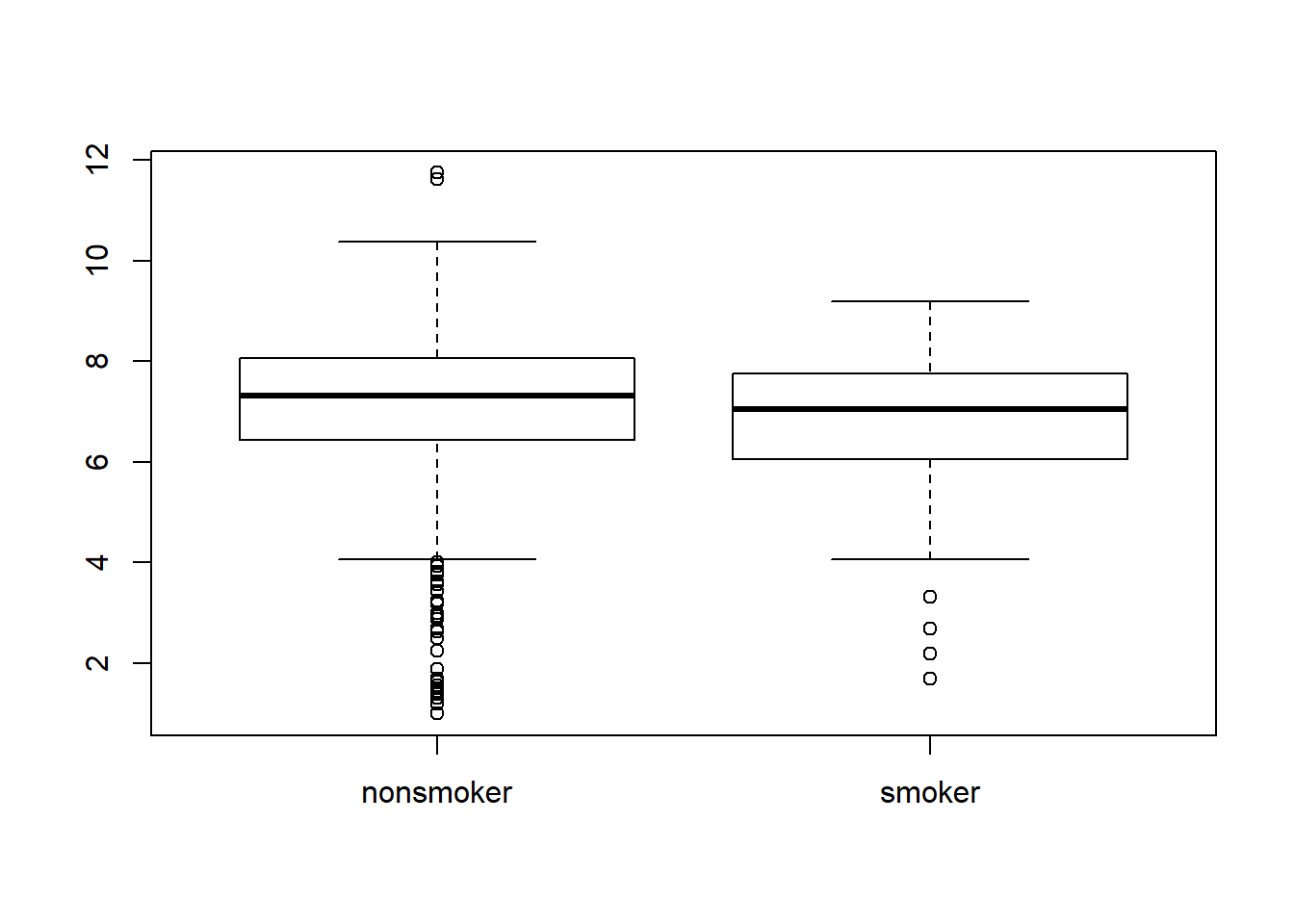The plot shows that the median weights are pretty close for both categories (smoker and non-smoker), though the median weight is slightly higher for non-smoking mothers. Both categories have some outliers, though there seem to be may more outliers for the non-smoking mothers. The box plots show how the medians of the two distributions compare, but we can also compare the means of the distributions using the following function to split the weight variable into the habit groups, then take the mean of each using the mean function. by(nc$weight, nc$habit, mean) ## nc$habit: nonsmoker
##  7.144273
## --------------------------------------------------------
## nc$habit: smoker ##  6.82873 There is an observed difference, but is this difference statistically significant? In order to answer this question we will conduct a hypothesis test . Inference 1. Check if the conditions necessary for inference are satisfied. Note that you will need to obtain sample sizes to check the conditions. You can compute the group size using the same by command above but replacing mean with length. by(nc$weight, nc$habit, length) ## nc$habit: nonsmoker
##  873
## --------------------------------------------------------
## nc$habit: smoker ##  126 The sample size is adequate for making inferences. In both cases (smoker and non-smoker), it is above 30, and less than 10% of the population of mothers. It is mentioned that the sample was randomly drawn. 1. Write the hypotheses for testing if the average weights of babies born to smoking and non-smoking mothers are different. H0: avg.weight.smoker - avg.weight.nonsmoker = 0 Ha: avg.weight.smoker - avg.weight.nonsmoker <> 0 Next, we introduce a new function, inference, that we will use for conducting hypothesis tests and constructing confidence intervals. inference(y = nc$weight, x = nc$habit, est = "mean", type = "ht", null = 0, alternative = "twosided", method = "theoretical") ## Warning: package 'BHH2' was built under R version 3.5.3 ## Response variable: numerical, Explanatory variable: categorical ## Difference between two means ## Summary statistics: ## n_nonsmoker = 873, mean_nonsmoker = 7.1443, sd_nonsmoker = 1.5187 ## n_smoker = 126, mean_smoker = 6.8287, sd_smoker = 1.3862 ## Observed difference between means (nonsmoker-smoker) = 0.3155 ## ## H0: mu_nonsmoker - mu_smoker = 0 ## HA: mu_nonsmoker - mu_smoker != 0 ## Standard error = 0.134 ## Test statistic: Z = 2.359 ## p-value = 0.0184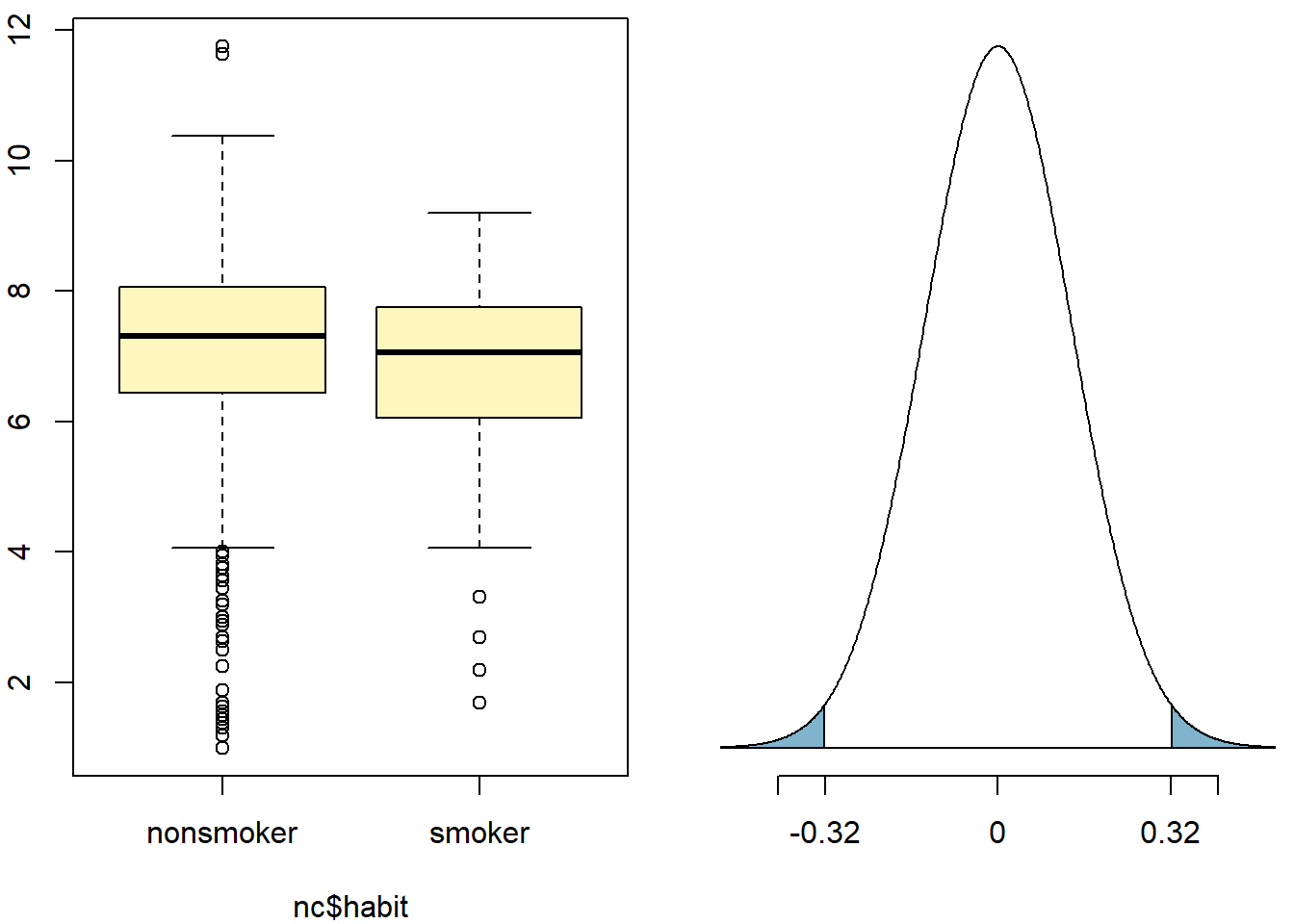Let’s pause for a moment to go through the arguments of this custom function. The first argument is y, which is the response variable that we are interested in: nc$weight. The second argument is the explanatory variable, x, which is the variable that splits the data into two groups, smokers and non-smokers: nc$habit. The third argument, est, is the parameter we’re interested in: "mean" (other options are "median", or "proportion".) Next we decide on the type of inference we want: a hypothesis test ("ht") or a confidence interval ("ci"). When performing a hypothesis test, we also need to supply the null value, which in this case is 0, since the null hypothesis sets the two population means equal to each other. The alternative hypothesis can be "less", "greater", or "twosided". Lastly, the method of inference can be "theoretical" or "simulation" based. 1. Change the type argument to "ci" to construct and record a confidence interval for the difference between the weights of babies born to smoking and non-smoking mothers. inference(y = nc$weight, x = nc$habit, est = "mean", type = "ci", null = 0, alternative = "twosided", method = "theoretical") ## Response variable: numerical, Explanatory variable: categorical ## Difference between two means ## Summary statistics: ## n_nonsmoker = 873, mean_nonsmoker = 7.1443, sd_nonsmoker = 1.5187 ## n_smoker = 126, mean_smoker = 6.8287, sd_smoker = 1.3862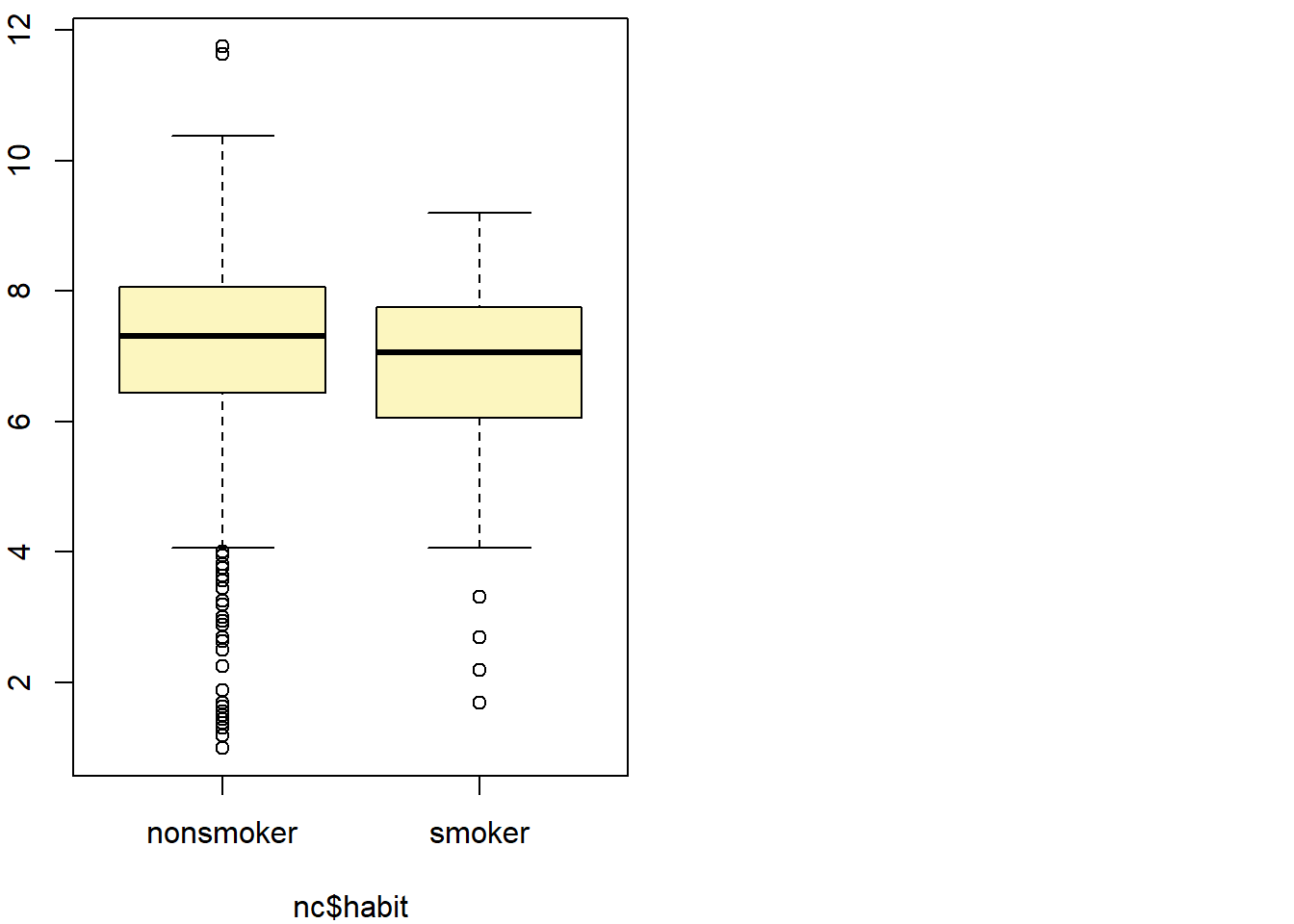## Observed difference between means (nonsmoker-smoker) = 0.3155 ## ## Standard error = 0.1338 ## 95 % Confidence interval = ( 0.0534 , 0.5777 ) By default the function reports an interval for ($$\mu_{nonsmoker} - \mu_{smoker}$$) . We can easily change this order by using the order argument: inference(y = nc$weight, x = nc$habit, est = "mean", type = "ci", null = 0, alternative = "twosided", method = "theoretical", order = c("smoker","nonsmoker")) ## Response variable: numerical, Explanatory variable: categorical ## Difference between two means ## Summary statistics: ## n_smoker = 126, mean_smoker = 6.8287, sd_smoker = 1.3862 ## n_nonsmoker = 873, mean_nonsmoker = 7.1443, sd_nonsmoker = 1.5187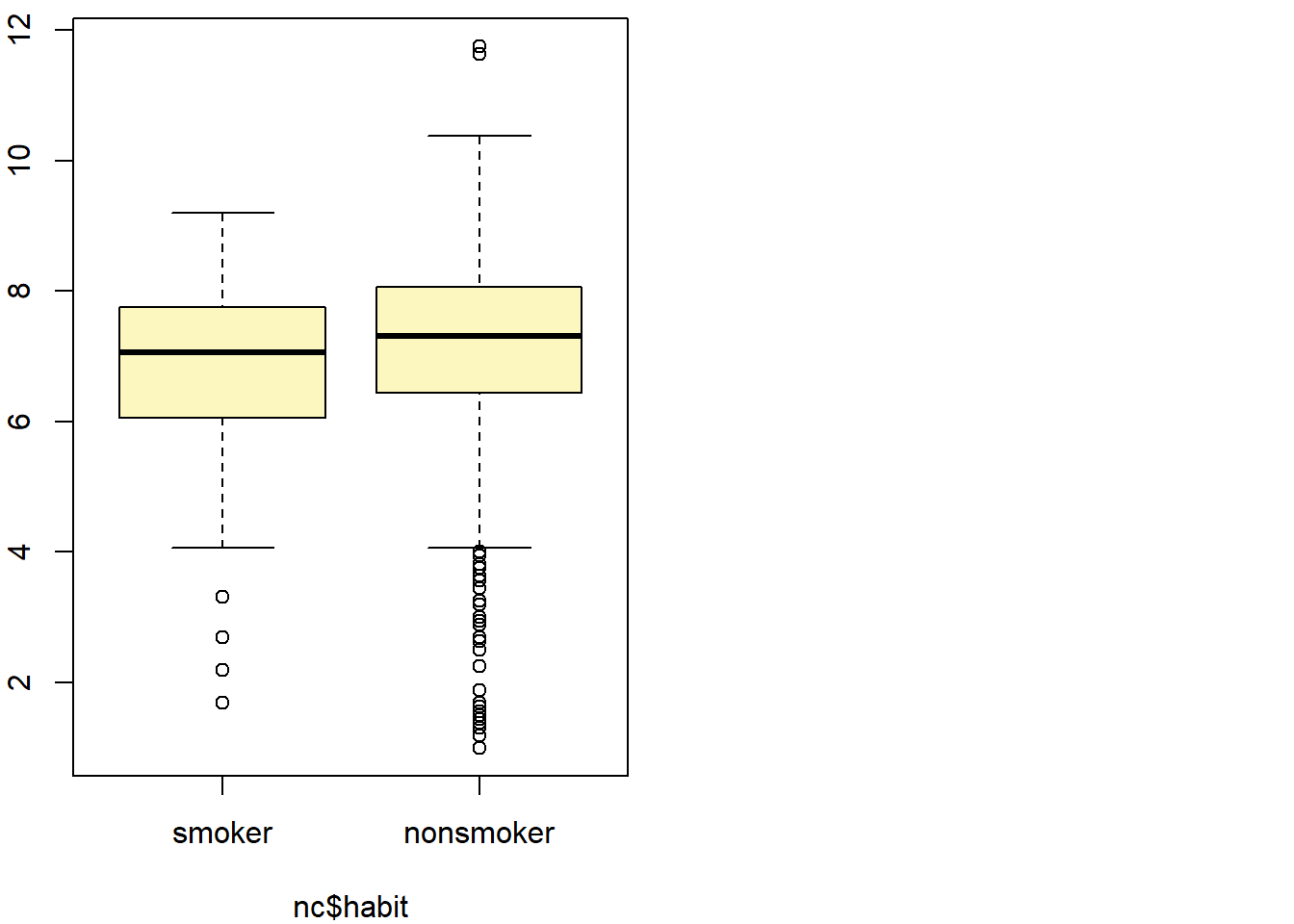## Observed difference between means (smoker-nonsmoker) = -0.3155 ## ## Standard error = 0.1338 ## 95 % Confidence interval = ( -0.5777 , -0.0534 ) On your own • Calculate a 95% confidence interval for the average length of pregnancies (weeks) and interpret it in context. Note that since you’re doing inference on a single population parameter, there is no explanatory variable, so you can omit the x variable from the function. inference(y = nc$weeks, est = "mean", type = "ci", null = mean(nc$weeks), alternative = "twosided", method = "theoretical" ) ## Single mean ## Summary statistics: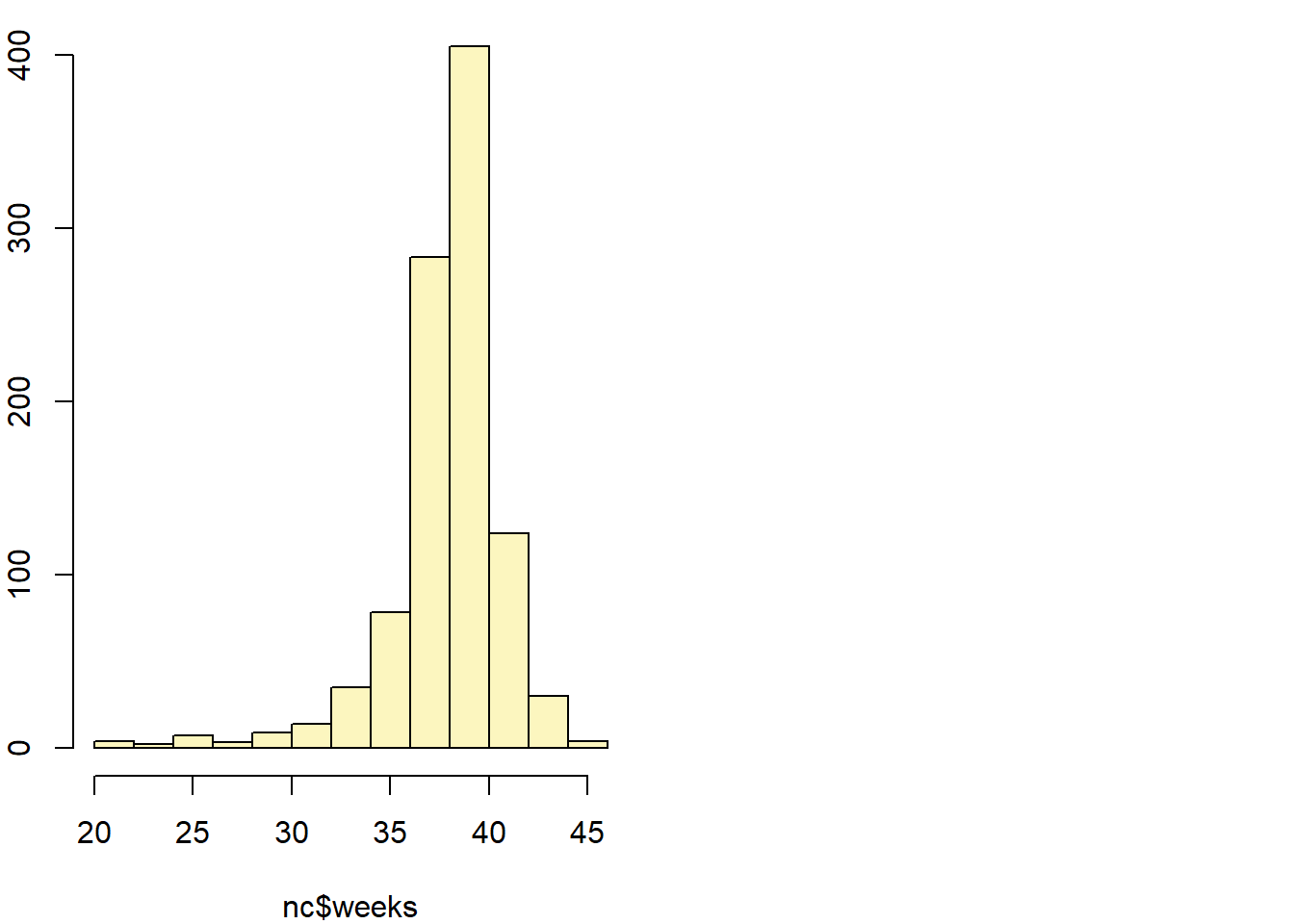## mean = 38.3347 ; sd = 2.9316 ; n = 998 ## Standard error = 0.0928 ## 95 % Confidence interval = ( 38.1528 , 38.5165 ) From the above, it can be seen that the 95% confidence interval for the average length of the pregnancy in the population is between 38.15 and 38.51 weeks. • Calculate a new confidence interval for the same parameter at the 90% confidence level. You can change the confidence level by adding a new argument to the function: conflevel = 0.90. inference(y = nc$weeks, est = "mean", type = "ci", null = mean(nc$weeks), alternative = "twosided", method = "theoretical", conflevel=0.90 ) ## Single mean ## Summary statistics:## mean = 38.3347 ; sd = 2.9316 ; n = 998 ## Standard error = 0.0928 ## 90 % Confidence interval = ( 38.182 , 38.4873 ) • Conduct a hypothesis test evaluating whether the average weight gained by younger mothers is different than the average weight gained by mature mothers. mean(nc$gained, na.rm = TRUE)
##  30.3258
nc.new<-subset(nc,is.na(nc$gained)==0, select=c(gained, mature)) by(nc.new$gained, nc.new$mature, mean) ## nc.new$mature: mature mom
##  28.7907
## --------------------------------------------------------
## nc.new$mature: younger mom ##  30.56043 H0: avg.gain.mature - avg.gain.younger = 0 Ha: avg.gain.mature - avg.gain.younger <> 0 • Now, a non-inference task: Determine the age cutoff for younger and mature mothers. Use a method of your choice, and explain how your method works. nc.age<-subset(nc, is.na(nc$mage)==0,select=c(mage, mature))
by(nc.age$mage, nc.age$mature, max)
## nc.age$mature: mature mom ##  50 ## -------------------------------------------------------- ## nc.age$mature: younger mom
##  34
by(nc.age$mage, nc.age$mature, min)
## nc.age$mature: mature mom ##  35 ## -------------------------------------------------------- ## nc.age$mature: younger mom
##  13

Based on the above, it can be seen that the maximum age for younger mothers is 34 years, and the minimum age for mature mothers is 35 years. So the cutoff is 35 years.

• Pick a pair of numerical and categorical variables and come up with a research question evaluating the relationship between these variables. Formulate the question in a way that it can be answered using a hypothesis test and/or a confidence interval. Answer your question using the inference function, report the statistical results, and also provide an explanation in plain language.
boxplot(nc$weight~nc$premie)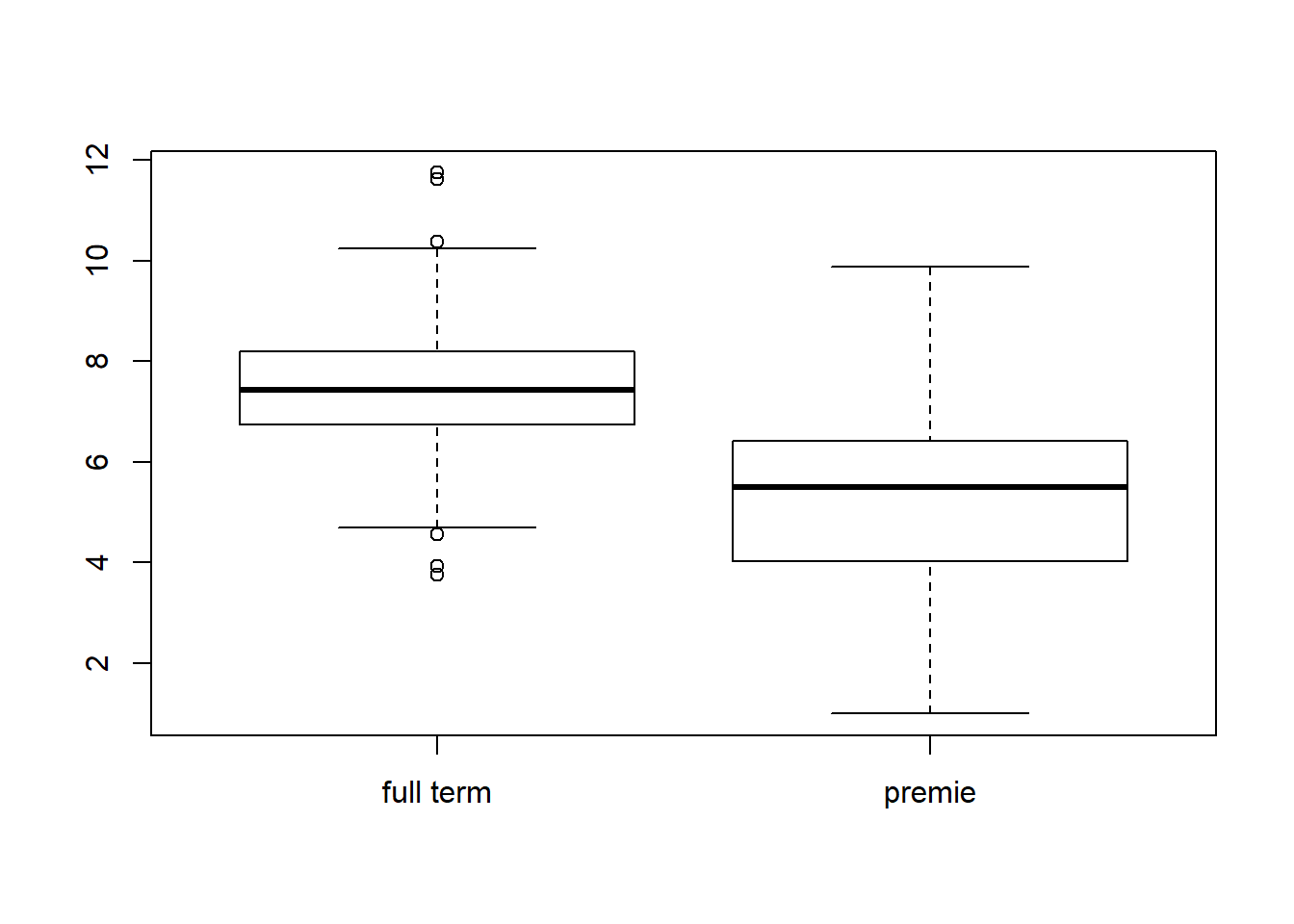H0: avg.weight.fullterm - avg.weight.premature = 0

Ha: avg.weight.fullterm - avg.weight.premature <> 0

The above hypotheses test whether the weight of the baby at birth is affected by whether it is full-term or pre-mature.

inference(y = nc$weight, x = nc$premie, est = "mean", type = "ci", null = 0,
alternative = "twosided", method = "theoretical")
## Response variable: numerical, Explanatory variable: categorical
## Difference between two means
## Summary statistics:
## n_full term = 846, mean_full term = 7.4594, sd_full term = 1.075
## n_premie = 152, mean_premie = 5.1284, sd_premie = 1.9696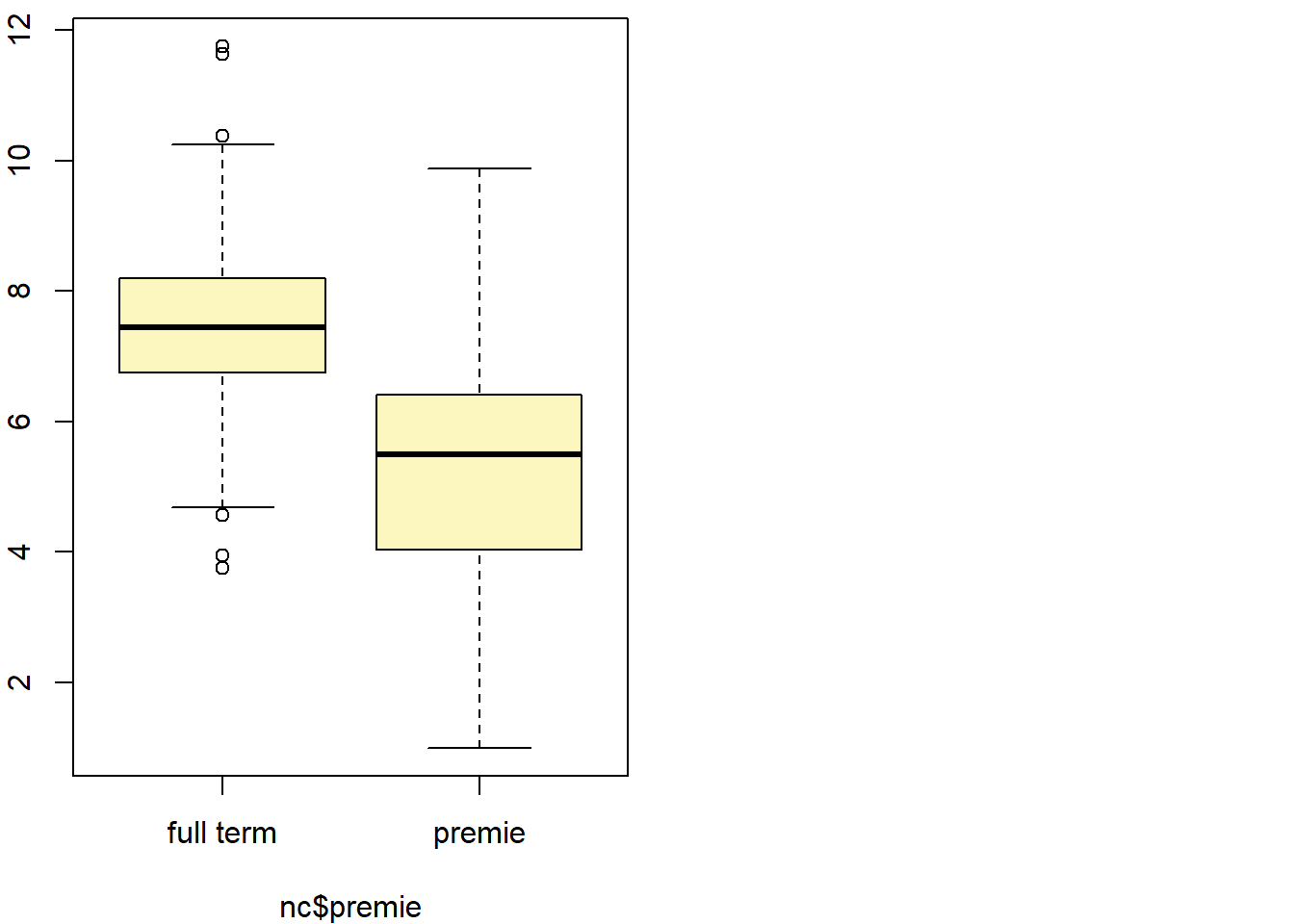## Observed difference between means (full term-premie) = 2.331
##
## Standard error = 0.164
## 95 % Confidence interval = ( 2.0096 , 2.6524 )
inference(y = nc$weight, x = nc$premie, est = "mean", type = "ht", null = 0,
alternative = "twosided", method = "theoretical")
## Response variable: numerical, Explanatory variable: categorical
## Difference between two means
## Summary statistics:
## n_full term = 846, mean_full term = 7.4594, sd_full term = 1.075
## n_premie = 152, mean_premie = 5.1284, sd_premie = 1.9696
## Observed difference between means (full term-premie) = 2.331
##
## H0: mu_full term - mu_premie = 0
## HA: mu_full term - mu_premie != 0
## Standard error = 0.164
## Test statistic: Z =  14.216
## p-value =  0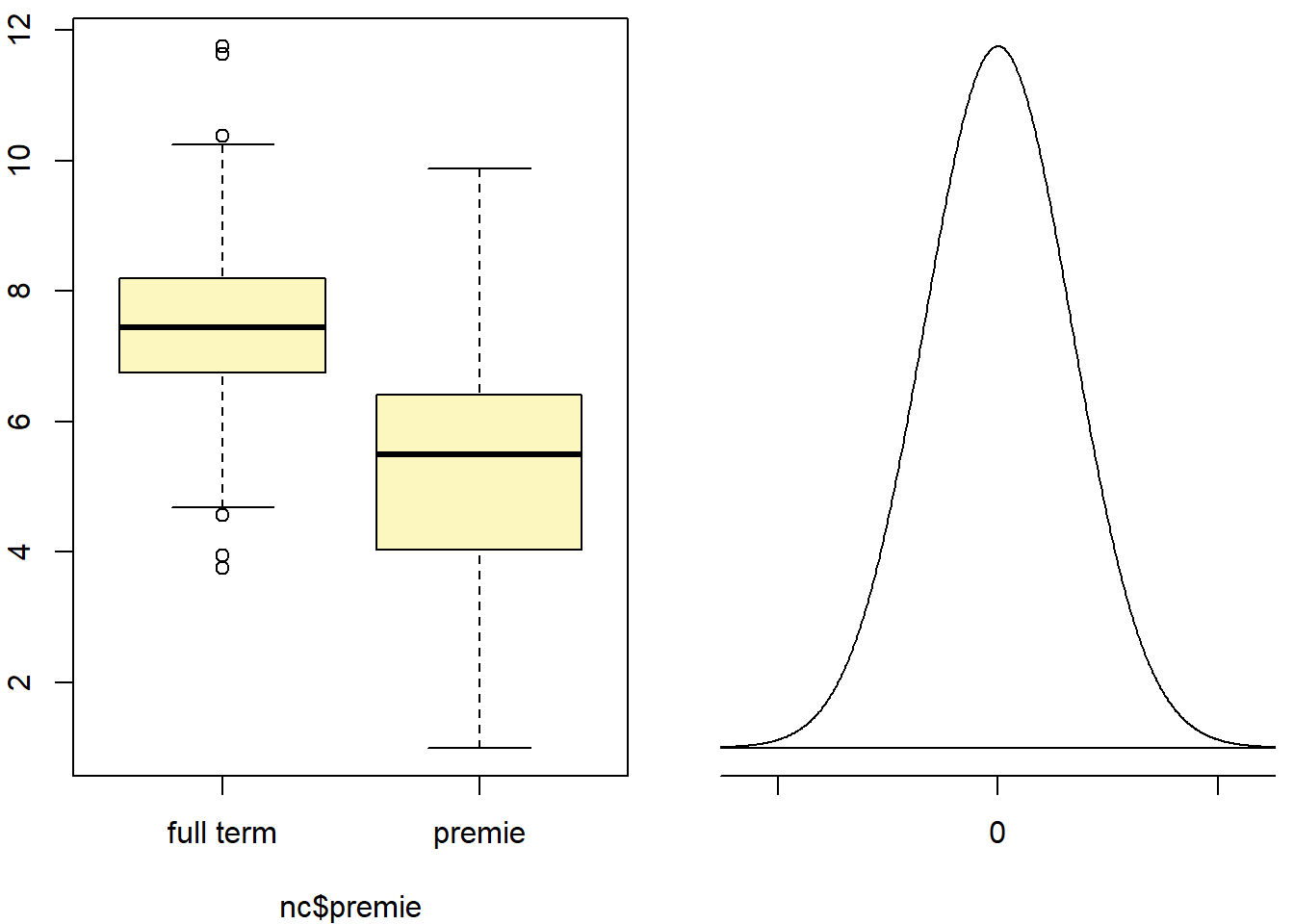Based on both hypothesis test and confidence intervals, the null hypothesis can be rejected at the 95% confidence level. The null value of 0 is not contained in the confidence interval, and the p-value associated with the test-statistic is 0.

This is a product of OpenIntro that is released under a Creative Commons Attribution-ShareAlike 3.0 Unported. This lab was adapted for OpenIntro by Mine Çetinkaya-Rundel from a lab written by the faculty and TAs of UCLA Statistics.